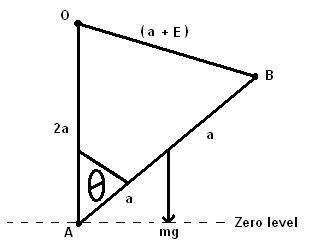# Stability physics problem

Just come across a question and I'm at a point where i see no further.

A uniform rod AB, of mass m and length 2a, is free to rotate in a vertical plane, about the end A. A light elastic string of modulus kmg and natural length a, has one and attached to B and the other end to a fixed point O which is vertically above A with OA = 2a. Show that when AB makes an angle $$\theta$$ with the downward vertical, the potential energy, V, of the system when the string is stretched is given by

$$V = mga[(4k - 1)\cos\theta -4k\cos\frac{\theta}{2}] + constant$$

I drew the following diagram:-I equated the GPE of the uniform rod to be
$$AB = -mg\cos\theta$$

And when it comes to calculating the energy of the elastic string i tried:

$$OC = \frac{\lambda x^2}{2a}$$

Where x is the extension in the string, which i calculated to be:

$$x = (2a\sin\theta - a)$$

So:
$$OB = \frac{kmg(2a\sin\theta - a)^2}{2a}$$

$$OB = \frac{kmga(4\sin^2\theta -4\sin\theta + 1)}{2}$$

$$OB = 2kmgasin^2\theta -2kmg\sin\theta + \frac{kmg}{2}$$

But since:
$$OB = \frac{kmg}{2}$$ is a constant, we can take it out of the equation.

Using the identity: $$\sin^2\theta = 2\sin\theta\cos\theta$$

$$4kmga\sin\theta\cos\theta -2kmga\sin\theta$$

However i can't get the 2nd term in the above equation to equal $$4kmga\cos\frac{\theta}{2}$$

Any help would be really appreciated.

Thank you.

Last edited:

Pyrrhus
Homework Helper
Hey Bob, how can you be sure the angle made by AB and OB is 90 degrees?

Hmm. Since the triangle OAB is isosceles, could i split it into 2 right angled triangles to get the length of the extension in terms of $$\cos\frac{\theta}{2}$$?

I'm not too clear why the sine of the angle theta can't just be used. Is there a fundamental flaw in my understanding of mathematics?

Thank you.

Just ran through it trying splitting the triangle and i got

$$OB = kmg(4a\cos\theta -4a\cos\frac{\theta}{2})$$

As this is equal to the GPE of the rod.

$$mga\cos\theta = kmg(4a\cos\theta -4a\cos\frac{\theta}{2})$$

$$V = mga[(4k - 1)\cos\theta - 4k\cos\frac{\theta}{2}]$$